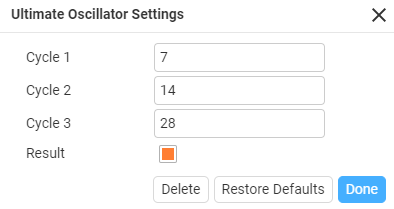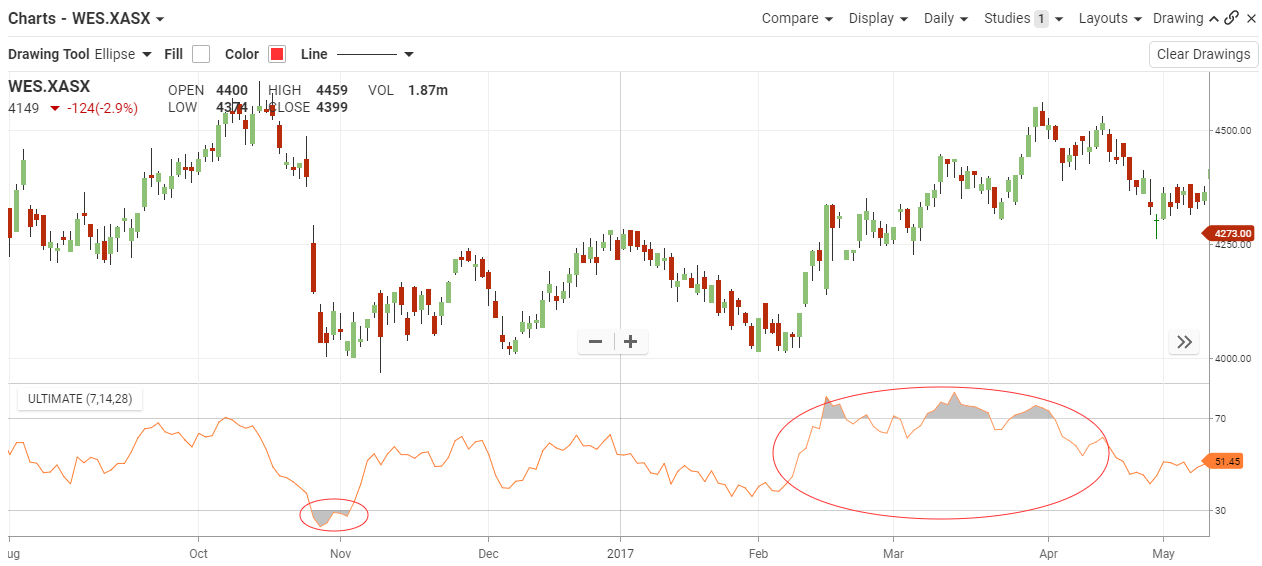## Ultimate Oscillator

#### The Basics

The Ultimate Oscillator is a technical analysis tool used to measure momentum across three varying timeframes. The problem with many momentum oscillators is that after a rapid advance or decline in price, they can form false divergence trading signals.

Indicator Type

Momentum Oscillator

Markets

All cash and futures, not options

Works Best

Trending markets

Formula

Almost all momentum oscillators generate signals based on bullish and bearish divergences. Therefore, frequent false signals based on these divergences would obviously be a problem. By including multiple timeframes, and weighting them accordingly the Ultimate Oscillator aims to cut down on these false signals

ParametersLength1
The length of the 1st time period. 7 is the Default.

Length2
The length of the 2nd time period. 14 is the Default.

Length3
The length of the 3rd time period. 28 is the Default.

#### Theory

This is a range-bound indicator, which means the value fluctuates between 0 and 100. Similar to the RSI, levels below 30 are deemed to be oversold, and levels above 70 are deemed to be overbought. Transaction signals are derived by finding situations where the price is going in opposite directions than the indicator. Once this divergence has been identified the trader will wait to confirm the transaction by using other technical indicators.

#### InterpretationWest Farmers had a sharp drop the at the end of October 2016, which bought it under the 30-line triggering oversold. It had choppy movement until February 2017, where the overbought started to rise along with the price. With the price movement it stayed overbought until April where it lost momentum and price ceased to its rise.

#### Maths

There are three steps to calculating the Ultimate Oscillator. This example uses 7, 14, 28 parameters:

1. Before calculating the Ultimate Oscillator, two variable need to be defined; Buying Pressure andTrue Range.

Buying Pressure (BP) = Close – Minimum (Lowest between Current Low or Previous Close)

True Range (TR) = Maximum (Highest between Current High or Previous Close) – Minimum (Lowest between Current Low or Previous Close)

1. The Ultimate Oscillator then uses these figures over three time periods:

Average7 = (7 Period BP Sum) / (7 Period TR Sum)

Average14 = (14 Period BP Sum) / (14 Period TR Sum)

Average28 = (28 Period BP Sum) / (28 Period TR Sum)

1. The final Ultimate Oscillator calculations can now be made:

UO =100 x [(4 x Average7)+(2 x Average14)+Average28]/(4+2+1)